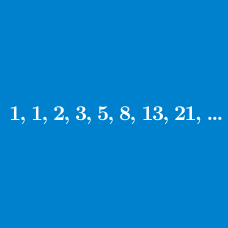Number Theory

# General Term Pattern Recognition

A general term of the sequence $-8, -7, -9, -5, -13, 3, \cdots$ can be expressed as $\displaystyle \frac{a-b^{n-1}}{3}$, where $a$ and $b$ are integers. What is the value of $ab$?

Which of the options represents the general term of the following sequence:

$1, 8, 27, 64, 125 \ldots ?$

Which of the options represents the general term of the following sequence:

$2, 5, 28, 257, 3126, \ldots$

Which of the options represents the general term of the following sequence:

$4, 6, 8, 10, 12 \ldots ?$

Which of the options represents the general term of the following sequence:

$5, 9, 13, 17, 21 \ldots ?$

×

Problem Loading...

Note Loading...

Set Loading...